Definition and Examples

Curve: A drawn path that connects two points and doesn't cross itself. It can be straight or make turns.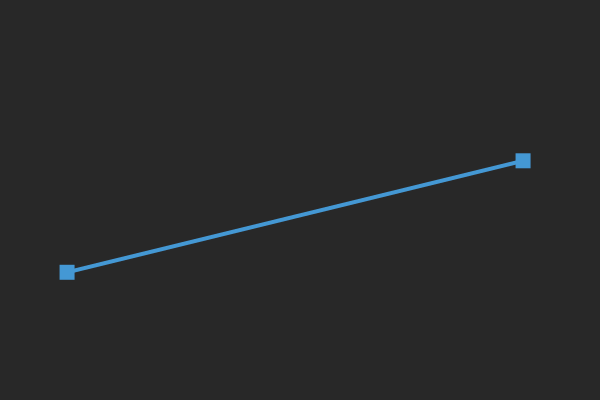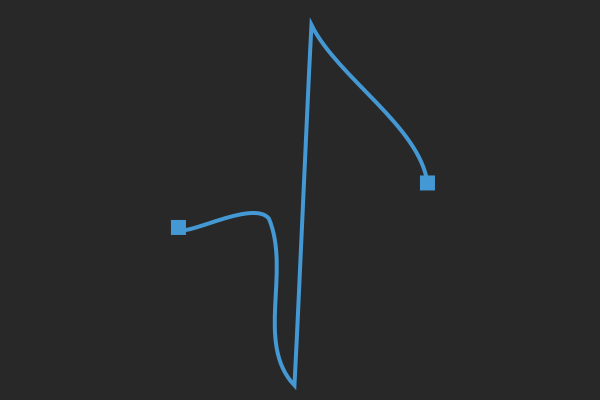S-Curve: A curve that when turned halfway around (upside down), looks exactly the same.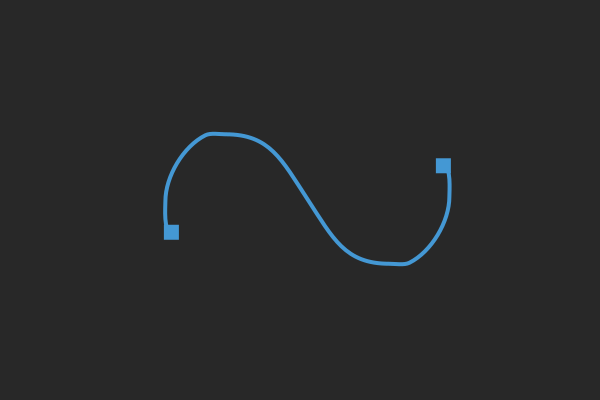Parallel Curves: Two curves exactly the same, where one can slide onto the other.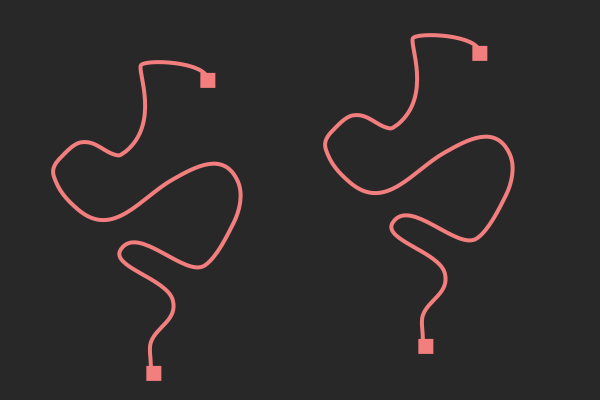Conway Tile: A tile made up of some S-curves and possibly 2 parallel curves. There are five different types, all guaranteed to tile the plane.

Tiling: Also called a tessellation, this is a filling of the plane by copies of tiles so that there are no gaps or overlaps.

Examples of tilings by Conway tiles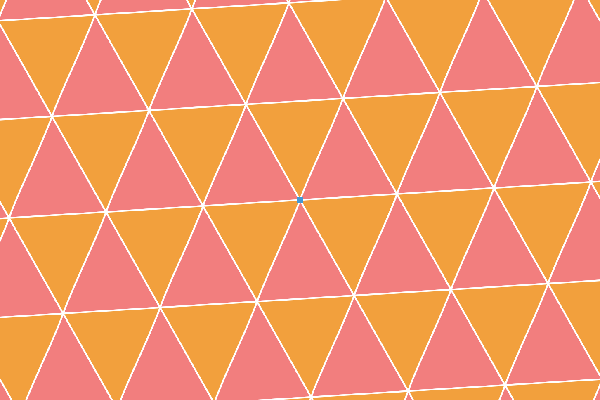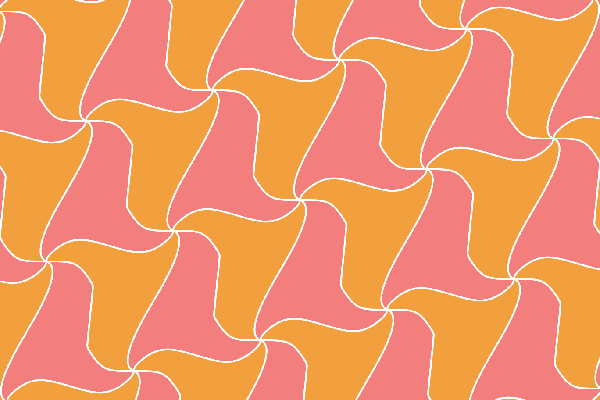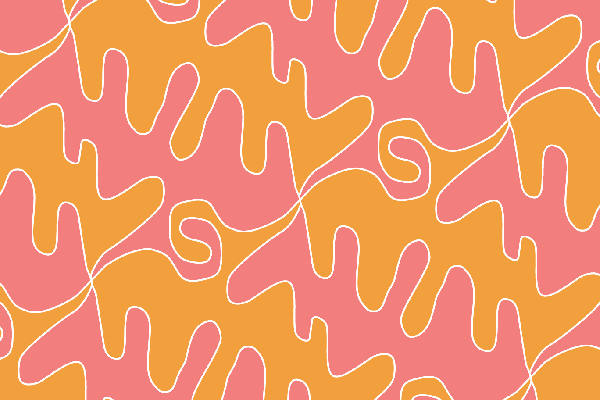Three connected S-curves.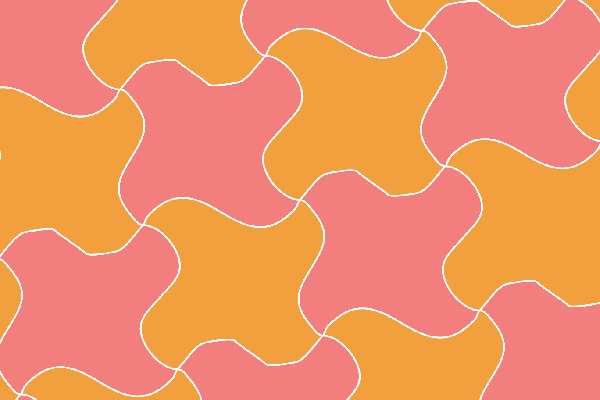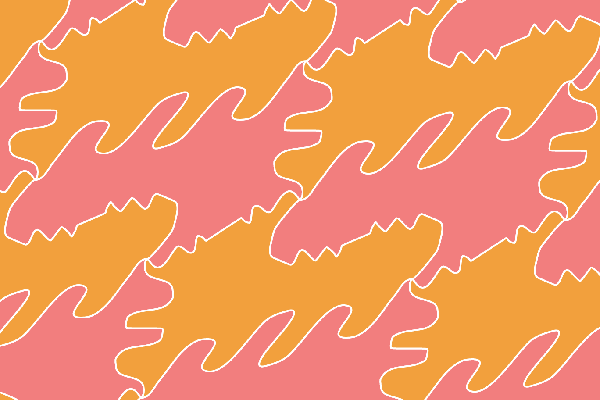Four connected S-curves.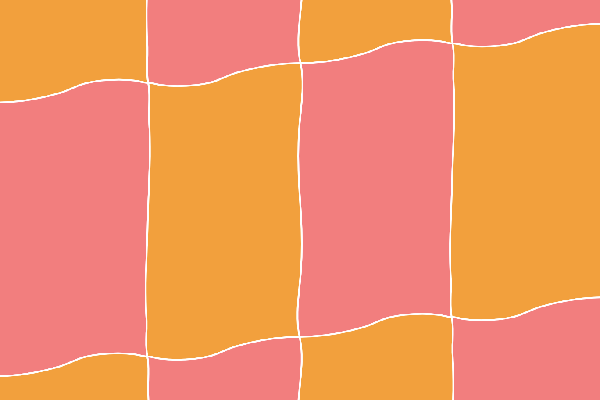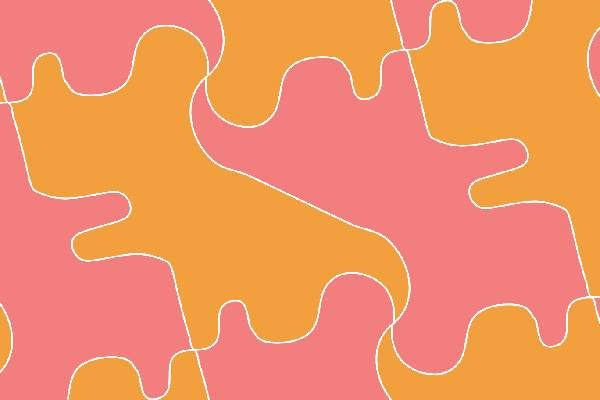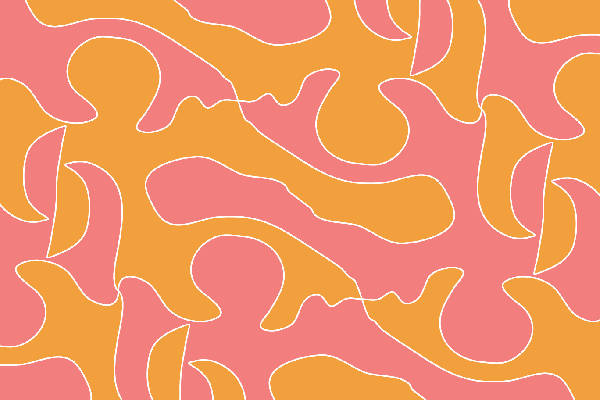Two parallel curves connected by two S-curves.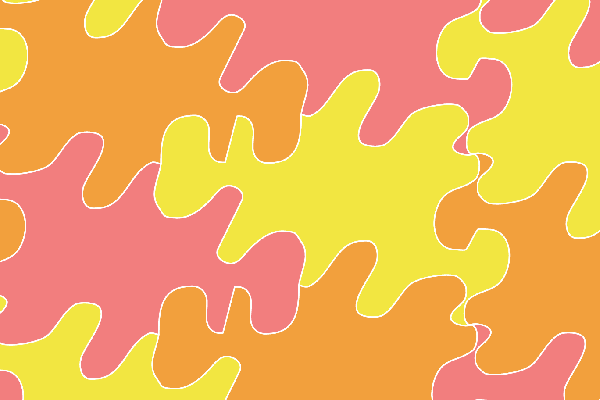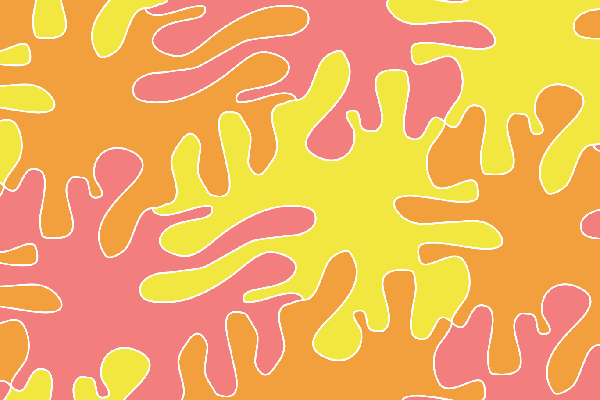Two parallel curves connected by three S-curves.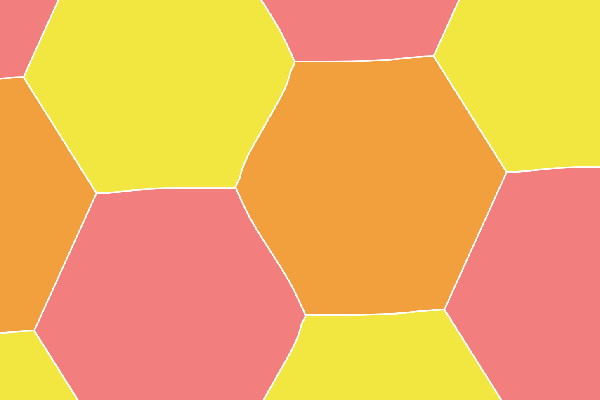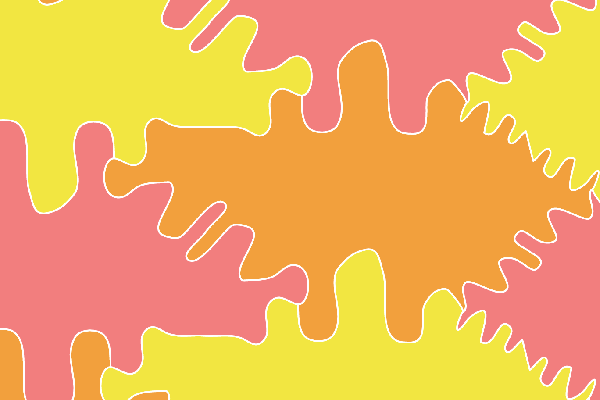Two parallel curves connected by four S-curves.